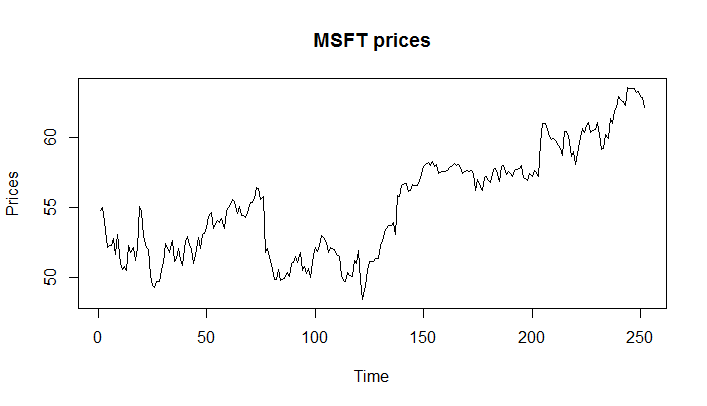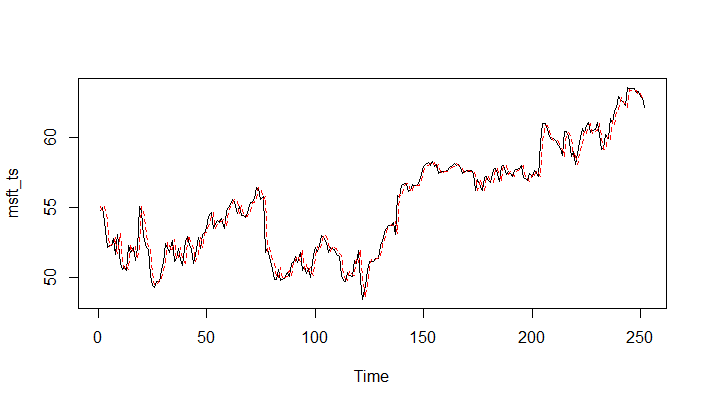# Estimating AutoRegressive (AR) Model in R

We will now see how we can fit an AR model to a given time series using the arima() function in R. Recall that AR model is an ARIMA(1, 0, 0) model.

We can use the arima() function in R to fit the AR model by specifying the order = c(1, 0, 0).

We will perform the estimation using the msft_ts time series that we created earlier in the first lesson. If you don't have the msft_ts loaded in your R session, please follow the steps to create it as specified in the first lesson.

Let's start by creating a plot of the original data using the plot.ts() function.

> plot.ts(msft_ts, main="MSFT prices", ylab="Prices")As of now we are not worried about whether an AR model is best suited for this data or not. Our objective is to understand the process of fitting the AR model to this data.

We will fit the AR model to this data using the following command:

msft_ar <- arima(msft_ts , order = c(1, 0, 0))


The output contains many things including the estimated slope (ar1), mean (intercept), and innovation variance (sigma^2) as shown below:

> msft_ar
Call:
arima(x = msft_ts, order = c(1, 0, 0))
Coefficients:
ar1  intercept
0.9815    56.2260
s.e.  0.0113     2.2359
sigma^2 estimated as 0.5891:  log likelihood = -292.55,  aic = 591.1
>


The msft_ar object also contains the residuals (εt ). Using the summary() function, you can see that the object contains a time series of residuals.

> summary(msft_ar) Length Class Mode coef 2 -none- numeric sigma2 1 -none- numeric var.coef 4 -none- numeric mask 2 -none- logical loglik 1 -none- numeric aic 1 -none- numeric arma 7 -none- numeric residuals 252 ts numeric call 3 -none- call series 1 -none- character code 1 -none- numeric n.cond 1 -none- numeric nobs 1 -none- numeric model 10 -none- list

You can extract the residuals using the residuals() function in R.

residuals <- residuals(msft_ar)

Once you find the residuals εt, the fitted values are just X̂t\=Xt−εt. In R, we can do it as follows:

msft_fitted <- msft_ts - residuals

We can now plot both the original and the fitted time series to see how close the fit is.

ts.plot(msft_ts) points(msft_fitted, type = "l", col = 2, lty = 2)### Calculating Residuals Manually from the Fitted Model (Advanced Content)

The AR(1) model is expressed as follows:

Xt = Ar * Xt-1 + εt

So,

εt = Xt - Ar * Xt-1

Where Ar is the estimated autoregressive part in the fitted model.

n=252 e=rep(1,n) e=0 for (t in (2 : n)){ e[t] = msft_ts [t]-coef(msft_ar )*msft_ts [t-1] }

Suppose the model was ARIMA(1,0,1)

εt = Xt - Ar * Xt-1 - Ma * Xt-1

> n=252

e=rep(1,n) e=0 for (t in (2 : n)){ e[t] = msft_ts [t]-coef(msft_ar )*msft_ts [t-1]-coef(msft_ar )*e[t-1] }

Get our Data Science for Finance Bundle for just $29$51.